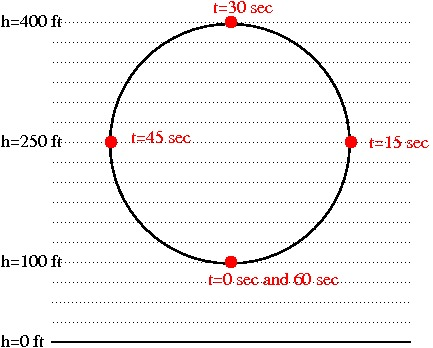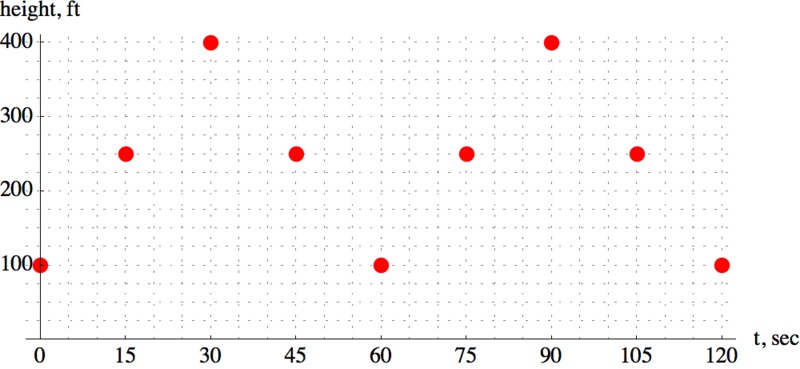# Model air plane acrobatics

Alignments to Content Standards: F-IF.B.4

A model airplane pilot is practicing flying her airplane in a big loop for an upcoming competition. At time $t=0$ her airplane is at the bottom of the loop 100 feet above the ground. The loop is a supposed to be a perfect circle, at its highest point the airplane is 400 feet above the ground, and it takes her 60 seconds to complete one loop. The airplane completes two loops and it is supposed to fly at a constant speed the entire time to score well in the competition.

1. The diagram below is a representation of the loop of the airplane. Draw in points that show where the airplane is every 15 seconds, starting at $t=0$. Assume that the plane is going around the circle in the counterclockwise direction.2. Make a table of values of the height of the airplane in feet above the ground as a function of time in seconds for the two loops.
3. Draw a graph of the height of the airplane during the two minute time interval on the axes below.## IM Commentary

This task could serve as an introduction to periodic functions and as a lead-in to sinusoidal functions. By visualizing the height of a plane that is moving along the circumference of a circle several times, students get the idea that output values of the height functions will repeat themselves after each complete revolution. They also connect the situation with key features on the graph, for example they interpret the midline and amplitude of the function as the height of the center of the circle and its radius.

When students draw the graph of the function, they will most likely plot the points that correspond to the height of the plane every quarter circle, i.e. every 15 seconds. When asked how to connect the points, they often draw line segments from point to point thereby creating a zig-zaging graph. This can lead to a very interesting discussion, especially if students have different ideas of how to finish the graph. Prompting students to find the height every 7.5 or 5 seconds and plotting those points as well is very eye-opening and leads to the shape of the desired sinusoidal function.

When going over the problem in class, the students (and the instructor) may want to combine parts (b) and (c), adding more entries to the table and graphing the corresponding points side by side.

If the task is used as an introduction to sinusoidal functions, it provides the need to define sine and cosine. So far students have not encountered functions that have wave like graphs. We now have the motivation to define new functions that behave in this way. Note that this example provides definitions of sine and cosine as a function of time, which provides a nice contextual segue into the definition of sine and cosine in terms of the radians.

The context of this task can be revisited throughout the material on sinusoidal functions. After the sine and cosine functions have been introduced, students can find formulas for the height of the plane as a function of time. Once we have a formula for the function, we can use it to solve an equation to find out when the plane is at a certain height above the ground.

Note that the context of the problem is not completely realistic. While it is possible to control a model airplane quite well, it is unlikely that the plane would travel in a perfect circle and at a constant speed. But making these simplifying assumptions is part of mathematical modeling and it enables us to learn a lot about the idealized situation.

## Solution

1. The red points show where the airplane will be every 15 seconds. Since the airplane is flying at a constant speed, it will fly along one fourth of the circumference of the circle every 15 seconds.2. We can estimate the height of the airplane at the red and blue points. The blue points show the position of the plane after 7.5, 22.5, 37.5 and 52.5 seconds.
time, seconds height, feet
0 100
7.5 140
15 250
22.5 360
30 400
37.5 360
45 250
52.5 140
60 100
3. If we plot the height of the airplane every 15 seconds we get the following graph.It is tempting to connect the points with straight lines segments. But adding the points corresponding to the height of the airplane in 7.5 second time intervals shows a more wave like shape:Looking back at the diagram of the loop we can see that the airplane gains and loses different amounts of height during equal time intervals.Reasoning in this way or by graphing more points we can draw the following graph for the entire time interval: